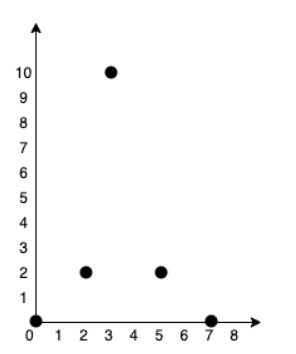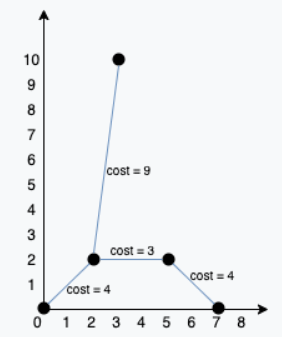##帐号 自动登录 找回密码 密码 立即注册
 搜索

# [学习笔记] Leetcode 1584. Min Cost to Connect All Points发表于 2020-9-16 21:25:21 | 显示全部楼层 |阅读模式

### 马上注册，结交更多好友，享用更多功能^_^

x
You are given an array points representing integer coordinates of some points on a 2D-plane, where points = [xi, yi].

The cost of connecting two points [xi, yi] and [xj, yj] is the manhattan distance between them: |xi - xj| + |yi - yj|, where |val| denotes the absolute value of val.

Return the minimum cost to make all points connected. All points are connected if there is exactly one simple path between any two points.

Example 1:Input: points = [[0,0],[2,2],[3,10],[5,2],[7,0]]
Output: 20
Explanation:We can connect the points as shown above to get the minimum cost of 20.
Notice that there is a unique path between every pair of points.
Example 2:

Input: points = [[3,12],[-2,5],[-4,1]]
Output: 18
Example 3:

Input: points = [[0,0],[1,1],[1,0],[-1,1]]
Output: 4
Example 4:

Input: points = [[-1000000,-1000000],[1000000,1000000]]
Output: 4000000
Example 5:

Input: points = [[0,0]]
Output: 0

Constraints:

1 <= points.length <= 1000
-106 <= xi, yi <= 106
All pairs (xi, yi) are distinct.

1. class UnionFind:
2.     def __init__(self, n: int):
3.         self.parent = list(range(n))
4.         self.size =  * n
5.
6.     def find(self, x):
7.         if self.parent[x] != x:
8.             self.parent[x] = self.find(self.parent[x])
9.         return self.parent[x]
10.
11.     def union(self, x, y):
12.         rx, ry = self.find(x), self.find(y)
13.         if rx == ry: return False
14.
15.         if self.size[rx] < self.size[ry]:
16.             rx, ry = ry, rx
17.
18.         self.parent[ry] = rx
19.         self.size[rx] += self.size[ry]
20.
21.         return True
22.
23. class Solution:
24.     def minCostConnectPoints(self, points: List[List[int]]) -> int:
25.         N = len(points)
26.         edges = []
27.         res = 0
28.
29.         for i in range(N):
30.             for j in range(i + 1, N):
31.                 distance = abs(points[i] - points[j])
32.                 distance += abs(points[i] - points[j])
33.
34.                 edges.append((distance, i, j))
35.
36.         edges.sort()
37.
38.         unionfind = UnionFind(N)
39.
40.         for edge in edges:
41.             dis, i, j = edge
42.             if unionfind.union(i, j):
43.                 res += dis
44.
45.         return res

### 本帖被以下淘专辑推荐:

 您需要登录后才可以回帖 登录 | 立即注册 本版积分规则 回帖后跳转到最后一页

GMT+8, 2020-9-28 02:00